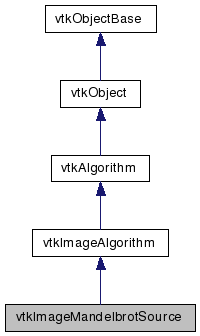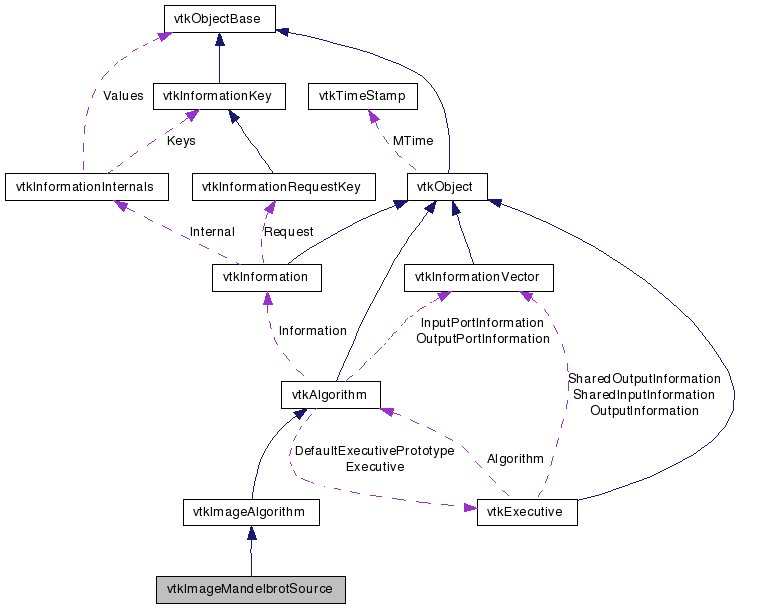# vtkImageMandelbrotSource Class Reference

`#include <vtkImageMandelbrotSource.h>`

Inheritance diagram for vtkImageMandelbrotSource:[legend]
Collaboration diagram for vtkImageMandelbrotSource:[legend]

## Detailed Description

Mandelbrot image.

vtkImageMandelbrotSource creates an unsigned char image of the Mandelbrot set. The values in the image are the number of iterations it takes for the magnitude of the value to get over 2. The equation repeated is z = z^2 + C (z and C are complex). Initial value of z is zero, and the real value of C is mapped onto the x axis, and the imaginary value of C is mapped onto the Y Axis. I was thinking of extending this source to generate Julia Sets (initial value of Z varies). This would be 4 possible parameters to vary, but there are no more 4d images :( The third dimension (z axis) is the imaginary value of the initial value.

Examples:
vtkImageMandelbrotSource (Examples)
Tests:
vtkImageMandelbrotSource (Tests)

Definition at line 40 of file vtkImageMandelbrotSource.h.

## Public Types

typedef vtkImageAlgorithm Superclass

## Public Member Functions

virtual const char * GetClassName ()
virtual int IsA (const char *type)
void PrintSelf (ostream &os, vtkIndent indent)
void CopyOriginAndSample (vtkImageMandelbrotSource *source)
void SetWholeExtent (int extent)
void SetWholeExtent (int minX, int maxX, int minY, int maxY, int minZ, int maxZ)
virtual int * GetWholeExtent ()
virtual void GetWholeExtent (int &, int &, int &, int &, int &, int &)
virtual void GetWholeExtent (int)
virtual void SetConstantSize (int)
virtual int GetConstantSize ()
virtual void ConstantSizeOn ()
virtual void ConstantSizeOff ()
void SetProjectionAxes (int x, int y, int z)
void SetProjectionAxes (int a)
virtual int * GetProjectionAxes ()
virtual void GetProjectionAxes (int &, int &, int &)
virtual void GetProjectionAxes (int)
virtual void SetOriginCX (double, double, double, double)
virtual void SetOriginCX (double)
virtual double * GetOriginCX ()
virtual void GetOriginCX (double &, double &, double &, double &)
virtual void GetOriginCX (double)
virtual void SetSampleCX (double, double, double, double)
virtual void SetSampleCX (double)
virtual double * GetSampleCX ()
virtual void GetSampleCX (double &, double &, double &, double &)
virtual void GetSampleCX (double)
void SetSizeCX (double cReal, double cImag, double xReal, double xImag)
double * GetSizeCX ()
void GetSizeCX (double s)
virtual void SetMaximumNumberOfIterations (unsigned short)
virtual unsigned short GetMaximumNumberOfIterations ()
void Zoom (double factor)
void Pan (double x, double y, double z)
virtual void SetSubsampleRate (int)
virtual int GetSubsampleRate ()

## Static Public Member Functions

static vtkImageMandelbrotSourceNew ()
static int IsTypeOf (const char *type)
static vtkImageMandelbrotSourceSafeDownCast (vtkObject *o)

## Protected Member Functions

vtkImageMandelbrotSource ()
~vtkImageMandelbrotSource ()
virtual int RequestData (vtkInformation *request, vtkInformationVector **inputVector, vtkInformationVector *outputVector)
virtual int RequestInformation (vtkInformation *, vtkInformationVector **, vtkInformationVector *)
double EvaluateSet (double p)

## Protected Attributes

int ProjectionAxes 
int WholeExtent 
double OriginCX 
double SampleCX 
unsigned short MaximumNumberOfIterations
double SizeCX 
int ConstantSize
int SubsampleRate

## Member Typedef Documentation

Reimplemented from vtkImageAlgorithm.

Definition at line 44 of file vtkImageMandelbrotSource.h.

## Constructor & Destructor Documentation

 vtkImageMandelbrotSource::vtkImageMandelbrotSource ( ) ` [protected]`

 vtkImageMandelbrotSource::~vtkImageMandelbrotSource ( ) ` [protected]`

## Member Function Documentation

 static vtkImageMandelbrotSource* vtkImageMandelbrotSource::New ( ) ` [static]`

Create an object with Debug turned off, modified time initialized to zero, and reference counting on.

Reimplemented from vtkAlgorithm.

 virtual const char* vtkImageMandelbrotSource::GetClassName ( ) ` [virtual]`

Reimplemented from vtkImageAlgorithm.

 static int vtkImageMandelbrotSource::IsTypeOf ( const char * name ) ` [static]`

Return 1 if this class type is the same type of (or a subclass of) the named class. Returns 0 otherwise. This method works in combination with vtkTypeRevisionMacro found in vtkSetGet.h.

Reimplemented from vtkImageAlgorithm.

 virtual int vtkImageMandelbrotSource::IsA ( const char * name ) ` [virtual]`

Return 1 if this class is the same type of (or a subclass of) the named class. Returns 0 otherwise. This method works in combination with vtkTypeRevisionMacro found in vtkSetGet.h.

Reimplemented from vtkImageAlgorithm.

 static vtkImageMandelbrotSource* vtkImageMandelbrotSource::SafeDownCast ( vtkObject * o ) ` [static]`

Reimplemented from vtkImageAlgorithm.

 void vtkImageMandelbrotSource::PrintSelf ( ostream & os, vtkIndent indent ) ` [virtual]`

Methods invoked by print to print information about the object including superclasses. Typically not called by the user (use Print() instead) but used in the hierarchical print process to combine the output of several classes.

Reimplemented from vtkImageAlgorithm.

 void vtkImageMandelbrotSource::SetWholeExtent ( int extent )

Set/Get the extent of the whole output Volume.

 void vtkImageMandelbrotSource::SetWholeExtent ( int minX, int maxX, int minY, int maxY, int minZ, int maxZ )

Set/Get the extent of the whole output Volume.

 virtual int* vtkImageMandelbrotSource::GetWholeExtent ( ) ` [virtual]`

Set/Get the extent of the whole output Volume.

 virtual void vtkImageMandelbrotSource::GetWholeExtent ( int & , int & , int & , int & , int & , int & ) ` [virtual]`

Set/Get the extent of the whole output Volume.

 virtual void vtkImageMandelbrotSource::GetWholeExtent ( int  ) ` [virtual]`

Set/Get the extent of the whole output Volume.

 virtual void vtkImageMandelbrotSource::SetConstantSize ( int ) ` [virtual]`

This flag determines whether the Size or spacing of a data set remain constant (when extent is changed). By default, size remains constant.

 virtual int vtkImageMandelbrotSource::GetConstantSize ( ) ` [virtual]`

This flag determines whether the Size or spacing of a data set remain constant (when extent is changed). By default, size remains constant.

 virtual void vtkImageMandelbrotSource::ConstantSizeOn ( ) ` [virtual]`

This flag determines whether the Size or spacing of a data set remain constant (when extent is changed). By default, size remains constant.

 virtual void vtkImageMandelbrotSource::ConstantSizeOff ( ) ` [virtual]`

This flag determines whether the Size or spacing of a data set remain constant (when extent is changed). By default, size remains constant.

 void vtkImageMandelbrotSource::SetProjectionAxes ( int x, int y, int z )

Set the projection from the 4D space (4 parameters / 2 imaginary numbers) to the axes of the 3D Volume. 0=C_Real, 1=C_Imaginary, 2=X_Real, 4=X_Imaginary

 void vtkImageMandelbrotSource::SetProjectionAxes ( int a ) ` [inline]`

Set the projection from the 4D space (4 parameters / 2 imaginary numbers) to the axes of the 3D Volume. 0=C_Real, 1=C_Imaginary, 2=X_Real, 4=X_Imaginary

Definition at line 68 of file vtkImageMandelbrotSource.h.

 virtual int* vtkImageMandelbrotSource::GetProjectionAxes ( ) ` [virtual]`

Set the projection from the 4D space (4 parameters / 2 imaginary numbers) to the axes of the 3D Volume. 0=C_Real, 1=C_Imaginary, 2=X_Real, 4=X_Imaginary

 virtual void vtkImageMandelbrotSource::GetProjectionAxes ( int & , int & , int & ) ` [virtual]`

Set the projection from the 4D space (4 parameters / 2 imaginary numbers) to the axes of the 3D Volume. 0=C_Real, 1=C_Imaginary, 2=X_Real, 4=X_Imaginary

 virtual void vtkImageMandelbrotSource::GetProjectionAxes ( int  ) ` [virtual]`

Set the projection from the 4D space (4 parameters / 2 imaginary numbers) to the axes of the 3D Volume. 0=C_Real, 1=C_Imaginary, 2=X_Real, 4=X_Imaginary

 virtual void vtkImageMandelbrotSource::SetOriginCX ( double , double , double , double ) ` [virtual]`

Imaginary and real value for C (constant in equation) and X (initial value).

 virtual void vtkImageMandelbrotSource::SetOriginCX ( double  ) ` [virtual]`

Imaginary and real value for C (constant in equation) and X (initial value).

 virtual double* vtkImageMandelbrotSource::GetOriginCX ( ) ` [virtual]`

Imaginary and real value for C (constant in equation) and X (initial value).

 virtual void vtkImageMandelbrotSource::GetOriginCX ( double & , double & , double & , double & ) ` [virtual]`

Imaginary and real value for C (constant in equation) and X (initial value).

 virtual void vtkImageMandelbrotSource::GetOriginCX ( double  ) ` [virtual]`

Imaginary and real value for C (constant in equation) and X (initial value).

 virtual void vtkImageMandelbrotSource::SetSampleCX ( double , double , double , double ) ` [virtual]`

Imaginary and real value for C (constant in equation) and X (initial value).

 virtual void vtkImageMandelbrotSource::SetSampleCX ( double  ) ` [virtual]`

Imaginary and real value for C (constant in equation) and X (initial value).

 virtual double* vtkImageMandelbrotSource::GetSampleCX ( ) ` [virtual]`

Imaginary and real value for C (constant in equation) and X (initial value).

 virtual void vtkImageMandelbrotSource::GetSampleCX ( double & , double & , double & , double & ) ` [virtual]`

Imaginary and real value for C (constant in equation) and X (initial value).

 virtual void vtkImageMandelbrotSource::GetSampleCX ( double  ) ` [virtual]`

Imaginary and real value for C (constant in equation) and X (initial value).

 void vtkImageMandelbrotSource::SetSizeCX ( double cReal, double cImag, double xReal, double xImag )

Just a different way of setting the sample. This sets the size of the 4D volume. SampleCX is computed from size and extent. Size is ignored when a dimension i 0 (collapsed).

 double* vtkImageMandelbrotSource::GetSizeCX ( )

Just a different way of setting the sample. This sets the size of the 4D volume. SampleCX is computed from size and extent. Size is ignored when a dimension i 0 (collapsed).

 void vtkImageMandelbrotSource::GetSizeCX ( double s )

Just a different way of setting the sample. This sets the size of the 4D volume. SampleCX is computed from size and extent. Size is ignored when a dimension i 0 (collapsed).

 virtual void vtkImageMandelbrotSource::SetMaximumNumberOfIterations ( unsigned short ) ` [virtual]`

The maximum number of cycles run to see if the value goes over 2

 virtual unsigned short vtkImageMandelbrotSource::GetMaximumNumberOfIterations ( ) ` [virtual]`

The maximum number of cycles run to see if the value goes over 2

 void vtkImageMandelbrotSource::Zoom ( double factor )

Convienence for Viewer. Pan 3D volume relative to spacing. Zoom constant factor.

 void vtkImageMandelbrotSource::Pan ( double x, double y, double z )

Convienence for Viewer. Pan 3D volume relative to spacing. Zoom constant factor.

 void vtkImageMandelbrotSource::CopyOriginAndSample ( vtkImageMandelbrotSource * source )

Convienence for Viewer. Copy the OriginCX and the SpacingCX. What about other parameters ???

 virtual void vtkImageMandelbrotSource::SetSubsampleRate ( int ) ` [virtual]`

Set/Get a subsample rate.

 virtual int vtkImageMandelbrotSource::GetSubsampleRate ( ) ` [virtual]`

Set/Get a subsample rate.

 virtual int vtkImageMandelbrotSource::RequestData ( vtkInformation * request, vtkInformationVector ** inputVector, vtkInformationVector * outputVector ) ` [protected, virtual]`

This is called by the superclass. This is the method you should override.

Reimplemented from vtkImageAlgorithm.

 virtual int vtkImageMandelbrotSource::RequestInformation ( vtkInformation * , vtkInformationVector ** , vtkInformationVector * ) ` [protected, virtual]`

Reimplemented from vtkImageAlgorithm.

 double vtkImageMandelbrotSource::EvaluateSet ( double p ) ` [protected]`

## Member Data Documentation

 int vtkImageMandelbrotSource::ProjectionAxes` [protected]`

Definition at line 124 of file vtkImageMandelbrotSource.h.

 int vtkImageMandelbrotSource::WholeExtent` [protected]`

Definition at line 127 of file vtkImageMandelbrotSource.h.

 double vtkImageMandelbrotSource::OriginCX` [protected]`

Definition at line 130 of file vtkImageMandelbrotSource.h.

 double vtkImageMandelbrotSource::SampleCX` [protected]`

Definition at line 132 of file vtkImageMandelbrotSource.h.

 unsigned short vtkImageMandelbrotSource::MaximumNumberOfIterations` [protected]`

Definition at line 133 of file vtkImageMandelbrotSource.h.

 double vtkImageMandelbrotSource::SizeCX` [protected]`

Definition at line 137 of file vtkImageMandelbrotSource.h.

 int vtkImageMandelbrotSource::ConstantSize` [protected]`

Definition at line 140 of file vtkImageMandelbrotSource.h.

 int vtkImageMandelbrotSource::SubsampleRate` [protected]`

Definition at line 142 of file vtkImageMandelbrotSource.h.

The documentation for this class was generated from the following file:

Generated on Sat Dec 27 13:30:17 2008 for VTK by1.5.6